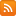CONTÁCTENOS - 91 575 78 24Estás en www.librosingenieria.com
Si no encuentra un libro lo buscamos por Ud.
91 575 78 24

CESTA DE LA COMPRA

Tiene 0 productos en su cesta Importe total: 0
Strutctural Analysis with finite element

Strutctural Analysis with finite element

Descripción

Structural Analysis with Finite Elements reveals the theory behind the finite element (FE) method as it relates to structural engineering and explains how to overcome commonly encountered problems and errors found in everyday structural modelling with finite element software.

Características

• ISBN: 9780727740939
• Páginas: 414
• Tamaño: 17x24
• Edición:
• Idioma: Inglés
• Año: 2010

Contenido Strutctural Analysis with finite element

Structural Analysis with Finite Elements reveals the theory behind the finite element (FE) method as it relates to structural engineering and explains how to overcome commonly encountered problems and errors found in everyday structural modelling with finite element software.

Drawing on nearly 20 years of experience as a structural engineer and FE software developer, Paolo Rugarli gives readers clear guidance on the fundamental principles of the FE method, demonstrating through practical examples how these principles apply to the kind of FE modelling that goes on every day in structural design departments.

Explaining computational methods from the software users' point of view, Structural Analysis with Finite Elements also points out the risks involved in using FE software packages, analysing typical errors and problems, with extensive reference to real world examples.

Key features of the book:

• Focuses on the practical structural engineering applications of FEM
• Goes back to first principles
• Gives readable, accessible and concise explanations
• Identifies common pitfalls that analysts encounter when devising structural models
• Applicable to all FEM software

Containing the theory and practice of finite element analysis, this book will prove a valuable guide for structural engineers and students worldwide.

1.Introduction

Overview

2.1.A Simile
2.2.The Principal Steps
2.2.1.Choosing the mathematical model
2.2.2.Choosing the most suitable type of finite element
2.2.3.Meshing
2.2.4.Assigning material and geometric characteristics
2.2.5.Assigning the constraints
2.2.7.Renumbering the nodes and creating the vectors
2.2.8.Assembly
2.2.9.Solution
2.2.10.Stress recovery
2.2.11.Output and interpretation of the results

3.Elasticity: Special Formulations

3.1.Introduction
3.2.The Energy Aspects of the Problem of Elasticity
3.3.Technical Beam Theory
3.3.1.Introduction, review of properties of plane areas
3.3.2.Elongation/?shortening
3.3.3.Bending and shear (Euler-Bernoulli)
3.3.4.Bending and shear (Timoshenko)
3.3.5.Torsion
3.3.6.Final considerations
3.4.Plane Stress
3.5.Plane Strain
3.6.Thin Plate (Germain-Kirchhoff)
3.7.Thick Plate (Mindlin-Reissner)
3.8.Axial Symmetry
3.9.Generic Solids
3.10.Summary

4.The Finite Element

4.1.Introduction
4.2.Inside the Finite Element
4.2.1.The problem
4.2.2.Element type, Element shape
4.2.3.The coordinate and connectivity tables
4.2.4.Connection
4.2.5.The basic hypotheses: The "Astrological principle"
4.3.Formal Developments: Finite Element Analytics
4.3.1.Congruity
4.3.2.Constitution
4.3.3.Energy aspects
4.3.4.Equilibrium
4.3.5.A simplified model for the structure
4.4.The Problem of Congruence and Compatibility
4.4.1.Classification of connections
4.5.Local and Global Systems
4.6.Calculating the Integrals
4.6.1.Introduction
4.6.2.Problems of geometry
4.6.3.Problems of integration

5.Assembly

5.1.Arriving at a Coherent Description
5.2.Nodal Displacement vectors: From Element to Structure (and Vice Versa)
5.3.Static Method
5.4.Energy Method
5.5.Remarks About the System of Solution Equations
5.6.1.Assembly of a simple structure
5.6.2.The static interpretation of the terms of the stiffness matrix
5.6.3.Energy interpretation of the Stiffness Matrix
5.6.4.Typical characteristics of the stiffness matrix
5.7.Heterogeneity of Elements

6.Actions

6.1.Introduction
6.2.Nodal Forces or Couples
6.3.1.The consistent approach
6.3.2.The lumped approach
6.3.3.Fixed end forces (Beams)
6.3.4.Which method?
6.3.5.Examples
6.5.Applied Actions and Constraint Reactions
6.6.2.Normative combinations: limit states and Allowable stresses
6.6.3.The principle of superposition of effects
6.7.Equivalent Static Analysis (Earthquake)
6.7.1.Overview of the problem
6.7.2.Conversion of actions into masses
6.7.3.Equivalent static actions

7.Constraints

7.1.Introduction
7.2.Real Constraints
7.2.1.Overview
7.2.2.Fixed constraints
7.2.3.Settling constraints
7.2.4.Kinematic constraints (Multipoint constraint)
7.2.5.Lability and Hypostaticity (Mechanisms)
7.2.6.Symmetrical constraints
7.3.Inactive Degrees of Freedom: Ad Hoc Formulations
7.4.Inactive Degrees of Freedom: Pseudo-Mechanism
7.4.1.Activated and inactivated pseudo-mechanism
7.4.2.Eliminating pseudo-mechanisms
7.4.3.Identifying pseudo-mechanisms numerically
7.5.Self-Equilibrating Structures

8.Solving and Stress Recovery

8.1.Solving
8.1.1.Brief review of methods of solving linear systems
8.1.2.Techniques for storing stiffness matrices
8.1.3.Renumbering
8.1.4.The user's point of view
8.2.Stress Recovery
8.2.1.How stress recovery works
8.2.2.Interpolation, extrapolation (local smoothing)
8.2.3.Interelement jumps
8.2.4.Global smoothing: The risks of cosmetics

9.Error Assessment

9.1.The Analyst and Error
9.2.Determination of Numerical Errors
9.2.1.Characteristics of convergence in the finite element method
9.2.2.Measurement of error: Residuals and constraint reactions
9.2.3.Unsmoothed stress maps
9.2.4.Significant digits: Truth and Myth
9.3.Looking for Modelling Errors
9.3.1.Overview
9.3.2.Formulation
9.3.3.The mesh
9.3.4.Double elements and double nodes
9.3.5.Cross section orientation and end releases
9.3.6.Symmetries and antisymmetries
9.3.7.Global and partial resultants
9.3.8.Deformed configurations
9.3.9.The fundamental role of engineering estimates
9.4.Software Errors

10.Structural Problems

10.1.Plane stress
10.2.Plane Strain
10.3.Axisymmetric Problems
10.4.Plate Problems
10.5.Shells
10.6.Solid Problems
10.7.Reticular Structures
10.8.Frames
10.9.Mixed Problems

11.The Most Common Finite Elements

11.1.Introduction
11.2.Truss Elements
11.2.1.2-node truss elements
11.2.2.3-node truss elements
11.3.Beam Elements
11.3.1.Overview
11.3.2.2-node Euler-Bernoulli elements
11.3.3.2-node Timoshenko elements
11.3.4.Elements with three or more nodes
11.4.Springs
11.5.Plane Stress and Plane Strain
11.5.1.3-node triangular element (Constant Strain triangle, CST)
11.5.2.The 6-node triangular element (Linear strain triangle)
11.5.3.4-node elements
11.5.5.Transition Elements
11.6.Membranes
11.7.Plate-Shell
11.7.1.Overview
11.7.2.DKT and DKQ elements (thin plates)
11.7.3.De Veubeke elements (thin plate)
11.7.4.Hughes elements (Thin or thick plates)
11.7.5.Dvorkin-Bathe elements (thin or thick plates, MITC4)
11.7.6.The "drilling DOF" problem
11.8.Solid Elements (Brief Remarks)
11.8.1.Overview
11.8.2.4-node tetrahedral element (tetra4)
11.8.3.10-node tetrahedral Element (tetra 10)
11.8.4.8-node isoparametric Element (hexa8)
11.8.5.20-node isoparametric element (hexa20)

12.Problems in Modelling

12.1.Rendering and Modelling
12.2.Typical Structures
12.2.1.Continuous beams
12.2.2.Trusses
12.2.3.Towers and Masts
12.2.4.Plane frames
12.2.5.Space frames
12.2.6.Masonry structures
12.2.7.Plates
12.2.8.Floor Slabs
12.2.9.Buildings with a bracing core or shear walls in reinforced concrete
12.2.10.Plinths
12.2.11.Reverse beams
12.2.12.Foundation slabs
12.2.13.Cylindrical tanks, shells
12.3.Connections
12.3.1.Homogeneous connections
12.3.2.Heterogeneous connections

13 The problem of Checking

13.1.Checkers and Solvers
13.2.Designers of Programs and Designers of Standards
1 3.2.1.How programable is a standard?
13.2.2.How far can a standard be extended?
13.2.3.The consistency of standards
13.2.4.Errors and tests
13.3.The Return of Arbitrariness

14.Appendix I: Notation and Review of Calculus
15.Appendix II: Review of the Theory of Elasticity
16.Appendix III: Principal Symbols
17.Appendix IV: Essential Glossary
18.References.

ACERCA DE LA EMPRESA